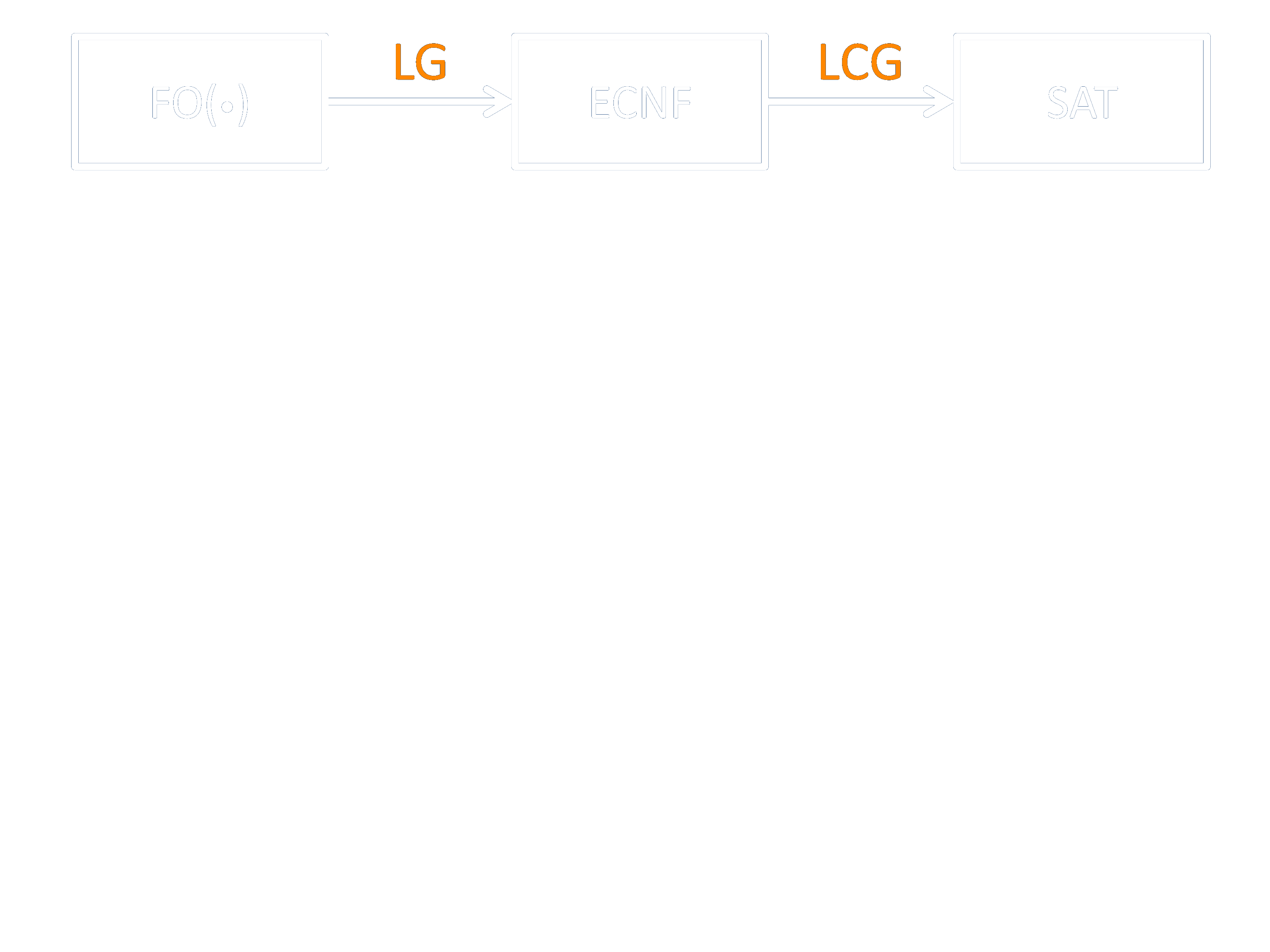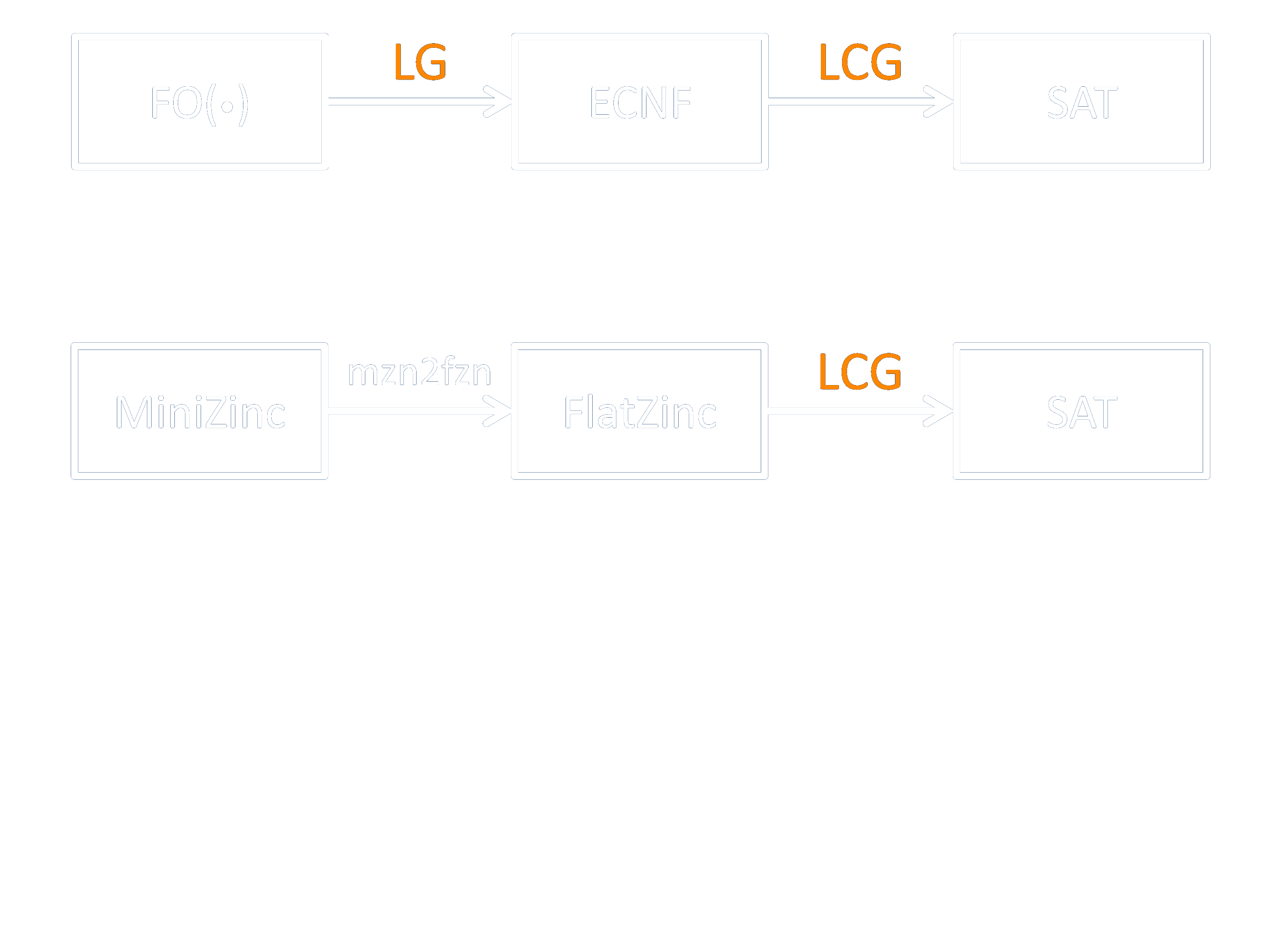# Lazy Model Expansion: Interleaving Grounding With Search

Broes de Cat, Marc Denecker, Peter J. Stuckey,
Maurice Bruynooghe, Bart Bogaerts

## FO(ID)

Typed first-order logic
Inductive Definitions

## Running Example: Sokoban

$\begin{array}{l} \left\{\begin{array}{l} \forall o, p: At(o,p,0)\leftarrow At_{in}(o,p)\\ \forall o, p, t: At(o, p ,t+1) \leftarrow Goto(o,p,t)\\ \forall o, p, t: At(o, p ,t+1) \leftarrow At(o,p,t) \land \lnot \exists p': Goto(o,p',t)\\ \end{array}\right\}\\ \left\{\begin{array}{l} \forall p, t: Goto(Sok,p,t) \leftarrow Move(p,t)\\ \forall b[Block], p, t: Goto(b,p,t) \leftarrow Push(b,p,t)\\ \forall b,p,p',t: Push(b,Next(p,p'),t) \\ \quad \leftarrow At(Sok,p,t) \wedge At(b,p',t)\wedge Move(p',t)\\ \end{array}\right\}\\ \forall p, t: Goto(p,t)\Rightarrow Empty(p,t) \vee \exists b[Block]: At(b,p,t)\\ \forall b, p, t: Push(b,p,t) \Rightarrow Empty(p,t)\\ \forall p, t: Empty(p,t) \Leftrightarrow \lnot \exists o: At(o,p,t)\\ \forall o, t: AtGoal(o,t) \Leftrightarrow At(o,Goal(o),t)\\ \forall t: GoalReached(t) \Leftrightarrow: \forall o: AtGoal(o,t) \end{array}$

## Model Expansion

Input:
• theory T
• partial interpretation S
• (output)vocabulary V

Output:
V-structure(s) M such that there is a M'
• M' is a model of T
• M' is more precise than S

Output: actions

Output: schedule

## Model Expansion: How?

First: ground to ECNF (extended CNF)
Then: solve the ground problem

## Problems

• Large domains
• Many nested quantifiers
• Infinite domains: impossible

## Solution

Don't make the entire grounding
Only ground what is necessary

## Lazy Grounding

Ground parts of the theory
based on the state of the solver

Some examples

## Universal Quantifications

$\forall b, p, t: Push(b,p,t) \Rightarrow Empty(p,t)$

$Push(b_1,p_1,0) \Rightarrow Empty(p_1,0)$

$Push(b_1,p_1,1) \Rightarrow Empty(p_1,1)$

$Push(b_1,p_1,2) \Rightarrow Empty(p_1,2)$

$\forall b, p, t: Push(b,p,t) \Rightarrow Empty(p,t)$

Actions are sparse

Solver state of $Push(b,p,t)$:
• unknown: do nothing
• false: do nothing
• true: instantiate quantification and ground

## Existential Quantifications

$\exists t: \mathit{GoalReached}(t)$

$\mathit{GoalReached}(0) \vee \mathit{GoalReached}(1) \vee \dots$
$\exists t[Time]: \mathit{GoalReached}(t)$

Rewrite to:
$\mathit{GoalReached} (0) \vee T$
$T \Rightarrow \exists t[\mathit{Time}\setminus\{0\}]: \mathit{GoalReached} (t)$

Solver state of $T$:
• unknown: do nothing
• false: do nothing
• true: rewrite to \begin{align*}T &\Rightarrow \mathit{GoalReached} (1) \vee T' \\ &\dots \end{align*}

## More General

$\forall x: P(x)\Rightarrow \phi(x)$
Delay on $P$:
only instantiate $x$ with values for which $P$ holds

$\exists x: \phi(x)$
only instantiate new values if all previous fail

## Lost Propagation

$\forall x: P(x) \Rightarrow \phi(x)$, delayed on $P$
$\phi(1) =\mathbf{f}$

Solution: use two watches
$\forall x: P(x) \land Q(x)\Rightarrow \phi(x)$
Delay on $P$ and $Q$

= generalisation of two watched literal scheme

## SoundNESS

Sound if every atom will eventually get a value

## Construction

= a deterministic recipe to extend
an interpretation such that
it satisﬁes certain constraints

$\forall x: P(x) \Rightarrow \phi(x)$
Default construction: make all $P(d)$'s false

$\forall x: P(x) \Leftrightarrow \phi(x)$
Definitional construction: assign $P(d)$ the value of $\phi(d)$

## Constructions

Constructions can be incompatible

Make all P(d)'s true
Assign P(d) the value of Q(d)

## Constructions

Constructions can be incompatible with solver state

Make all P(d)'s true
P(1) = false

## Lazy Grounding

• Split theory in
• Ground theory Tg
• High-level (delayed) theory Td
• For every sentence in Td
• find construction compatible with
• all other constructions
• the state of the solver
• If impossible: ground partially
• for relevant instantiations
• Solver searches model of T g

## Lazy Grounding

Afterwards, combine model of Tg   with
constructions for Td to obtain a model of T

## Lazy Grounding (in general)

• Very general framework
• Based on definitions and justifications

## Definitions

All (inductive) definitions that occur in practice are total:

For every assignment to their parameters,
there is exactly one assignment to the defined
symbols such that the definition is satisfied

Hence, definitions are constructions

## DefinitionS

Grounding of definitions can be delayed on
all defined symbols:

For a rule $\forall \bar{x}: P(\bar{x})\leftarrow \varphi$:
Delay on $P(\bar{x})$:
• Unknown: do nothing
• Otherwise: ground rule
• for relevant instantiation

= Dynamic Top-Down Grounding

## Implementation

Implemented in $\textrm{IDP}^3$

## Experiments

$$\require{color}$$ $\definecolor{myorange}{RGB}{255,153,0} \newcommand{\fancyprint}{\color{myorange}{#1}} \begin{array}{l|ccc|ccc} benchmark & \textrm{IDP} & \textrm{lazy} & \textrm{ASP} & \textrm{IDP} & \textrm{lazy} & \textrm{ASP}\\ \hline \text{Sokoban} &9 &5 &\fancyprint{10} & 370 &1538 &\fancyprint{22} \\ \text{Disj. Scheduling} &4 &\fancyprint{10} &2 &1933 &\fancyprint{92} &2400 \\ \text{Packing} &9 &\fancyprint{10} &1 &352 &\fancyprint{51} &2704 \\ \text{Labyrinth} &\fancyprint{6} &5 &\fancyprint{6} &1286 &1851 &\fancyprint{1015} \\ \text{Reachability} &1 &\fancyprint{10} &4 &2706 &\fancyprint{7} &1807 \\ \text{St. Marriage} &0 &\fancyprint{10} &5 &3000 &\fancyprint{350} &1563 \\ \text{Graph Colouring} &\fancyprint{7} &3 &4 &\fancyprint{1013} &2103 &1842 \end{array}$

## Lazy Clause Generation

• Fits in lazy grounding framework
• Richer language
• Lazy grounding also captures mzn2fzn## Lazy Clause Generation

• Fits in lazy grounding framework
• Richer language
• Lazy grounding also captures mzn2fzn## Lazy Clause Generation

• Fits in lazy grounding framework
• Richer language
• Lazy grounding also captures mzn2fzn## LCG, IP and LG

$A+B=C$

• LCG
• dedicated sum propagator
• lazily add learned clause, e.g., $A\geq 4 \wedge B\geq 5\Rightarrow C\geq 9$
• triggers on changes of $A$, $B$ and $C$
• IP
• Represent propagations in high level language
• E.g., whenever (for some $d,d'$) $A\geq d$ and $B\geq d'$, propagate $C\geq d+d'$
• Automatically obtain propagator
• LG
• LG on IP representation ($\forall d, d': A\geq d \wedge B\geq d' \Rightarrow C\geq d+d'$)
• = LCG behaviour!

## Incremental Grounding

• special case of lazy grounding

## Reference

Broes de Cat, Marc Denecker, Peter J. Stuckey, Maurice Bruynooghe: Lazy Model Expansion: Interleaving Grounding with Search. J. Artif. Intell. Res. (JAIR) 52: 235-286 (2015)